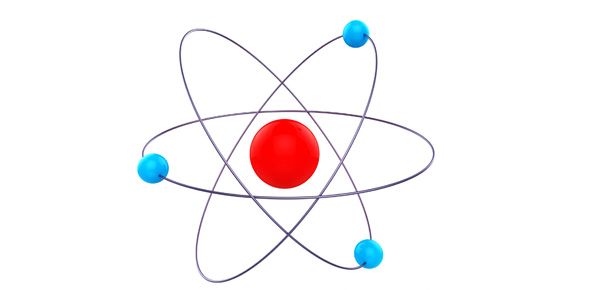# Chem Mastery Formula Mass Set 2

3 QuestionsSettingsRelated Topics
• 1.
What is the approximate molecular/formula mass of the following compound: Ni(OH)2
• A.

91

• B.

93

• C.

95

• D.

97

• E.

99

• 2.
What is the approximate molecular/formula mass of the following compound: (NH4)2SO3
• A.

106

• B.

108

• C.

110

• D.

112

• E.

114

• F.

116

• 3.
What is the approximate molecular/formula mass of the following compound: CaCl2.2H2O
• A.

146

• B.

147

• C.

148

• D.

149

• E.

150